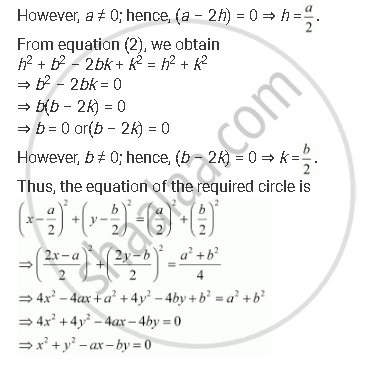CBSE (Arts) Class 11CBSE
Share
Notifications

View all notifications
Books Shortlist
Your shortlist is empty

# Find the Equation of the Circle Passing Through (0, 0) and Making Intercepts a and B on the Coordinate Axes. - CBSE (Arts) Class 11 - Mathematics

Login
Create free account

Forgot password?

#### Question

Find the equation of the circle passing through (0, 0) and making intercepts and b on the coordinate axes.

#### Solution

Let the equation of the required circle be (x – h)2 + (y – k)2 = r2.

Since the circle passes through (0, 0),

(0 – h)2 + (0 – k)2 = r2

⇒ h2 + k2 = r2

The equation of the circle now becomes (x – h)2 + (y – k)2 = h2 + k2.

It is given that the circle makes intercepts and b on the coordinate axes. This means that the circle passes through points (a, 0) and (0, b). Therefore,

(a – h)2 + (0 – k)2 = h2 + k2 … (1)

(0 – h)2 + (b – k)2 = h2 + k2 … (2)

From equation (1), we obtain

a2 – 2ah + h2 + k2 = h2 + k2

⇒ a2 – 2ah = 0

⇒ a(a – 2h) = 0

⇒ a = 0 or (a – 2h) = 0Is there an error in this question or solution?

#### APPEARS IN

Solution Find the Equation of the Circle Passing Through (0, 0) and Making Intercepts a and B on the Coordinate Axes. Concept: Concept of Circle.
S